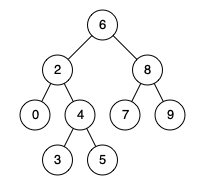# Tree - 235. Lowest Common Ancestor of a Binary Search Tree

235. Lowest Common Ancestor of a Binary Search Tree

Given a binary search tree (BST), find the lowest common ancestor (LCA) of two given nodes in the BST.

According to the definition of LCA on Wikipedia: “The lowest common ancestor is defined between two nodes p and q as the lowest node in T that has both p and q as descendants (where we allow a node to be a descendant of itself).”

Given binary search tree:  root = [6,2,8,0,4,7,9,null,null,3,5]Example 1:

``````Input: root = [6,2,8,0,4,7,9,null,null,3,5], p = 2, q = 8
Output: 6
Explanation: The LCA of nodes 2 and 8 is 6.

``````

Example 2:

``````Input: root = [6,2,8,0,4,7,9,null,null,3,5], p = 2, q = 4
Output: 2
Explanation: The LCA of nodes 2 and 4 is 2, since a node can be a descendant of itself according to the LCA definition.

Note:

- All of the nodes' values will be unique.
- p and q are different and both values will exist in the BST.

``````

go：

``````/**

* Definition for TreeNode.
* type TreeNode struct {
*     Val int
*     Left *ListNode
*     Right *ListNode
* }
*/
func lowestCommonAncestor(root, p, q *TreeNode) *TreeNode {
if root == nil || root == p || root == q {
return root
}

if root.Val > p.Val && root.Val > q.Val {   // pq都在左子树中
return lowestCommonAncestor(root.Left, p, q)
} else if root.Val < p.Val && root.Val < q.Val { // pq都在右子树中
return lowestCommonAncestor(root.Right, p, q)
} else { // pq一个在一边，LCA一定是当前“root节点”
return root
}
}
``````

Nothing just happens, it's all part of a plan.